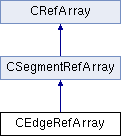﻿ C++: CEdgeRefArray Class Reference

CEdgeRefArray Class Reference

CEdgeRefArray Class Reference

`#include <xsi_edge.h>`

## Class Description

Array of Edge objects.

Warning:
This specialized array is returned by PolygonFace::GetEdges and PolygonMesh::GetEdges, it is not meant to be created and modified in user-defined functions. If you want to add and remove arbitrary items to a collection, you must use a CRefArray instead.
PolygonMesh::GetEdges, PolygonFace::GetEdges
Example:
```                using namespace XSI;
Application app;
Model root = app.GetActiveSceneRoot();

X3DObject myCube;
root.AddGeometry( L"Cube", L"MeshSurface", L"", myCube );

PolygonMesh mesh( myCube.GetActivePrimitive().GetGeometry() );

CEdgeRefArray edges(mesh.GetEdges());

app.LogMessage( L"Number of edges: " +
CValue(edges.GetCount()).GetAsText() );
```
Inheritance diagram for CEdgeRefArray:List of all members.

## Public Member Functions

CEdgeRefArray ()
CEdgeRefArray (const CEdgeRefArray &in_array)
CRef  GetItem (LONG in_index) const
CRef  GetItem (const CString &in_name) const
LONG  GetCount () const
bool  IsValid () const
CVertexRefArray  GetNeighborVertices (LONG in_lDistance=1) const
CEdgeRefArray  GetNeighborEdges (LONG in_lDistance=1) const
CPolygonFaceRefArray  GetNeighborPolygons (LONG in_lDistance=1) const
CEdgeRefArray  GrowNeighborEdges (LONG in_lDistance=1) const
CDoubleArray  GetCreaseArray () const
CBoolArray  GetIsHardArray () const

## Constructor & Destructor Documentation

 CEdgeRefArray ( )

Constructor.

 CEdgeRefArray ( const CEdgeRefArray & in_array )

Copy constructor.

Parameters:
 in_array constant CEdgeRefArray reference object.

## Member Function Documentation

 CRef GetItem ( LONG in_index ) const `[virtual]`

Returns the Edge object at the specified index in the array.

Parameters:
 in_index The index of the Edge.
Returns:
A reference to the specified Edge object.

Reimplemented from CSegmentRefArray.

 CRef GetItem ( const CString & in_name ) const `[virtual]`

Returns the Edge object in the array matching the specified name.

Parameters:
 in_name The name of the Edge.
Returns:
A reference to the specified Edge object.

Reimplemented from CSegmentRefArray.

 LONG GetCount ( ) const `[virtual]`

Gets the number of Edges in the array.

Returns:
The number of Edge objects.

Reimplemented from CSegmentRefArray.

 bool IsValid ( ) const `[virtual]`

Returns true if this array is a valid object or false otherwise.

Returns:
true if valid, false otherwise.

Reimplemented from CSegmentRefArray.

 CVertexRefArray GetNeighborVertices ( LONG in_lDistance = `1` ) const

Returns an array of Vertex neighbors within a given distance.

Parameters:
 in_lDistance Value Represents the degree of neighborhood (eg., degree=2 for an edge means its adjacent vertices plus the adjacent vertices of the adjacent vertices).
Returns:
An array of Vertex objects
 CEdgeRefArray GetNeighborEdges ( LONG in_lDistance = `1` ) const

Returns an array of Edge neighbors within a given distance.

Parameters:
 in_lDistance Value representing the degree of neighborhood (eg., degree=2 for an edge means its adjacent vertices plus the adjacent vertices of the adjacent vertices).
Returns:
An array of Edge objects
 CPolygonFaceRefArray GetNeighborPolygons ( LONG in_lDistance = `1` ) const

Returns an array of PolygonFace neighbors within a given distance.

Parameters:
 in_lDistance Value representing the degree of neighborhood (eg., degree=2 for an edge means its adjacent vertices plus the adjacent vertices of the adjacent vertices).
Returns:
An array of PolygonFace objects
 CEdgeRefArray GrowNeighborEdges ( LONG in_lDistance = `1` ) const

Returns an array of Edge objects on the geometry that are adjacent to the Edge objects contained in the array, within a given distance.

Parameters:
 in_lDistance Value representing the degree of neighborhood (eg., degree=2 for an edge means its adjacent vertices plus the adjacent vertices of the adjacent vertices).
Returns:
An array of Edge objects
 CDoubleArray GetCreaseArray ( ) const

Returns an array of crease values for all edges contained in this edge array.

Returns:
An array of crease values (doubles).
Since:
3.5.1
 CBoolArray GetIsHardArray ( ) const

Returns an array of boolean values indicating whether each edge in the array is hard or not.

Returns:
An array of boolean values.
Since:
3.5.1

The documentation for this class was generated from the following file: